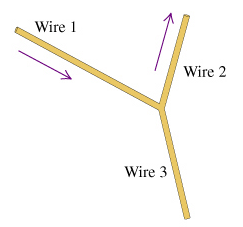# Problem: The magnitudes of the current density and the diameters for wires 1 and 2 are given in the table. The current directions are indicated by the arrows.WireCurrent density(A/mm2)Diameter(mm)14.02.125.73.0A) Find the Current I3 in wire 3. Express your answer in amperes to two significant figures. Call current out of the junction positive and current into the junction negative.B) Find the magnitude of the current density J3 in wire 3. The diameter of wire 3 is 1.5mm. Express your answer in amperes per square millimeter to two significant figures.

🤓 Based on our data, we think this question is relevant for Professor Ghimire's class at UCONN.

###### FREE Expert Solution

Current density,

$\overline{){\mathbf{J}}{\mathbf{=}}\frac{\mathbf{I}}{\mathbf{A}}}$

Where I is the current and A is the area.

Area,

$\overline{){\mathbf{A}}{\mathbf{=}}{\mathbf{\pi }}{{\mathbf{\left(}}\frac{\mathbf{d}}{\mathbf{2}}{\mathbf{\right)}}}^{{\mathbf{2}}}}$

Where d is the diameter.

A)

Assume that I3 enters the junction.

d1 = 2.1 mm

d2 = 3.0 mm

J1 = 4.0 A/mm2

J2 = 5.7 A/mm2###### Problem DetailsThe magnitudes of the current density and the diameters for wires 1 and 2 are given in the table. The current directions are indicated by the arrows.

 Wire Current density(A/mm2) Diameter(mm) 1 4.0 2.1 2 5.7 3.0

A) Find the Current I3 in wire 3. Express your answer in amperes to two significant figures. Call current out of the junction positive and current into the junction negative.

B) Find the magnitude of the current density J3 in wire 3. The diameter of wire 3 is 1.5mm. Express your answer in amperes per square millimeter to two significant figures.